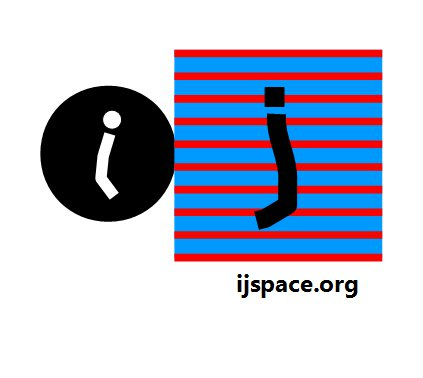Duality in j-space 15th March 2016 "In short, the time appears to have arrived, to attempt to unify the corpuscular and undulatory approaches in an attempt to reveal the fundamental nature of the quantum." - Louis Broglie in "On the Theory of Quanta".   "No interaction takes place between different light quanta (when gravitation is disregarded), but the interaction between light quanta and matter is responsible for the phenomena of absorption, emission and dispersion." - Heisenberg in "Principles of Quantum Theory". We will confine the discussion of duality to the measurements performed in a discrete measurement space or j-space.  Let us evaluate our problem of the path AB an observer ObsC wished to travel.  Initially ObsC is stuck, because in true j-space each point is different from each other and there is nothing in common.  Then VT-Symmetry is assumed and ObsC is able to define two points A and B with the measurement capacity (v/c ≈ 1) and therefore path AB is possible.     Please note that we have defined the points A and B based on an observer with maximum capacity.  The path AB is traveled by different observers and their measurements constitute different probability distributions.  These probability distributions vary from a delta function (capacity v/c ≈ 1), to a very noisy (capacity v/c << 1) probability distribution.     The duality we are interested in context to j-space, is the comparison between the continuous description and the discrete description of the path traveled by an observer.  We will like to understand if they represent the same event or if they are different from each other.     Consider two paths A-B and C-D requiring different efficiencies to complete measurements.  The points A and B can be measured by a high-efficiency observer ObsC only.  The points C and D can be measured by the low-efficiency observer ObsM as well as ObsC.  A low efficiency observer is likely to spend a lot more resources* traveling a path than a high efficiency observer.     To compare we need a unit of efficiency. Let us define it by the amount of resources used up to travel path A-B by the high efficiency observer ObsC (v/c ≈ 1) in a single measurement (zero-entropy measurement). We give it a symbol "ĥ"**.  Then the resources needed by the low efficiency observer to travel path C-D will be an integral multiple of  ĥ.  Why an integral multiple?  Because for ObsM the path AB'B can not exist hence no fraction of  ĥ is allowed.Remember we do not have any knowledge of energy or matter in j-space.  Instead we have a measurement which essentially is an interaction.  In Hamilton's anharmonic coordinates the interaction (the unit-point), itself is defined by a true-duality described by the axes OX and OY in OXY.  However we are not keen neither on the description of the measurement metric itself nor on the interpretation of the interaction in the metric itself.  We are trying to develop a skeleton we can cover with flesh afterwards.     All we know is that for a zero-entropy path a measurement metric, is not needed neither is the wave-particle duality.  The reason being that the points A, B and the zero-entropy path connecting them, are identically measured to the last detail and therefore they together represent a single point for ObsM.  For us this represents true discreteness.***      Therefore in j-space the wave propagation describes an entropic path or equivalently a path with variation with respect to the time-axis.  The point-representation and the wave-representation are not equivalent in j-space.  The wave-representation will follow only after "ĥ" is established and the time-axis is rolled out.    The discussion so far is required before we try to understand the conventional wave-particle duality and the importance of Quantum Mechanics. * The "resources required" will translate into the observer's metric as the "momentum required". For a high efficiency observer (v/c ≈ 1), the energy = pc where p is the observer momentum and c is the maximum velocity available to the observer in his/her measurement metric. ** The unit of efficiency "ĥ" , will have different interpretations for different observers and their respective measurement metrics.  More discussion on the correlation between ĥ and entropy of the measurements can be found here. *** Think topologically. Previous Blogs:   A Paradox   Nutshell-2015 Chiral Symmetry Sigma-z and I Spin Matrices Rationale behind Irrational Numbers The Ubiquitous z-Axis Majorana ZFC Axioms Set Theory Nutshell-2014 Knots in j-Space Supercolliders Force Riemann Hypothesis Andromeda Nebula Infinite Fulcrum Cauchy and Gaussian Distributions Discrete Space, b-Field & Lower Mass Bound Incompleteness II The Supersymmetry The Cat in Box The Initial State and Symmetries Incompleteness I Discrete Measurement Space The Frog in Well Visual Complex Analysis The Einstein Theory of RelativityBest viewed with Internet Explorer